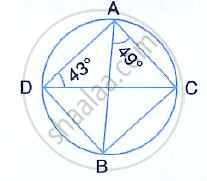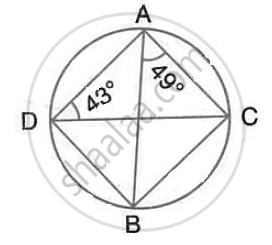Share

Calculate: (I) ∠Cdb, (Ii) ∠Abc, (Iii) ∠Acb - ICSE Class 10 - Mathematics

ConceptChord Properties - Chords Equidistant from the Center Are Equal (Without Proof)

Question

Calculate:
(i) ∠CDB, (ii) ∠ABC, (iii) ∠ACBSolutionHere,
∠CDB = ∠BAC = 49°
∠ABC =∠ADC = 43°
(Angle subtend by the same chord on the circle are equal)
By angle – sum property of a triangle,
∠ACB = 180°- 49° -43°= 88°

Is there an error in this question or solution?

Video TutorialsVIEW ALL 

Solution Calculate: (I) ∠Cdb, (Ii) ∠Abc, (Iii) ∠Acb Concept: Chord Properties - Chords Equidistant from the Center Are Equal (Without Proof).
S